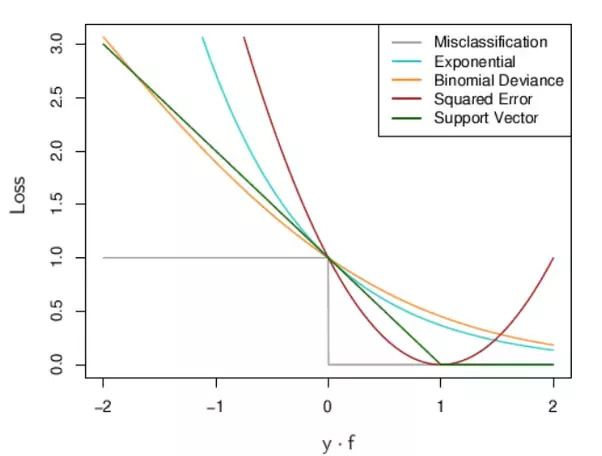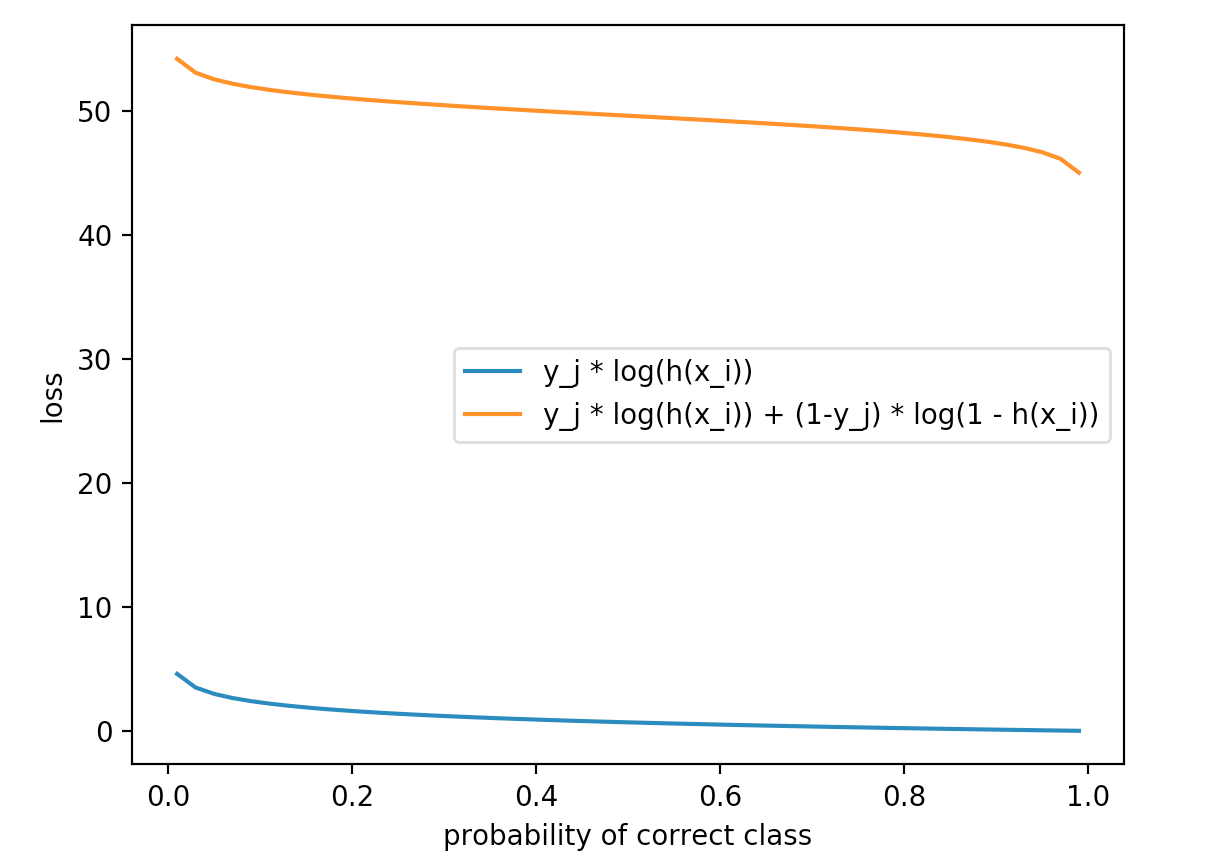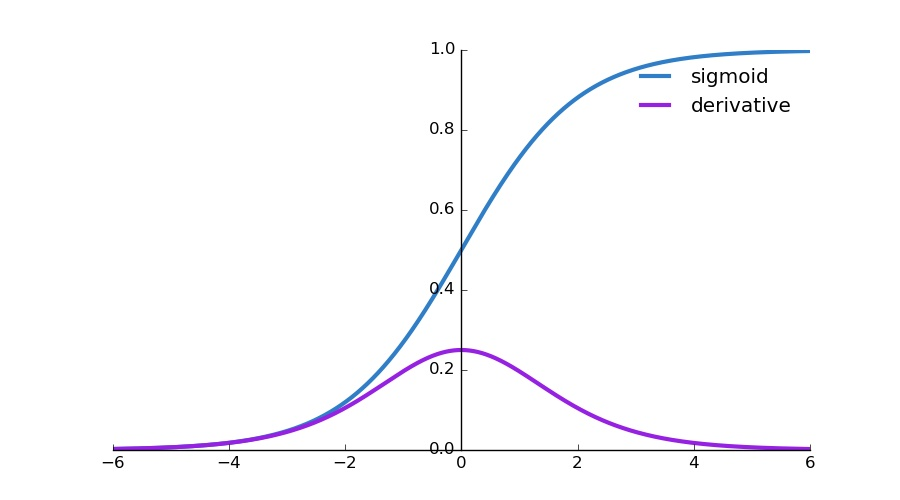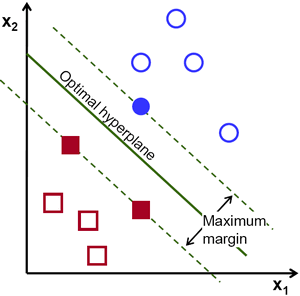# Picking Loss Functions - A comparison between MSE, Cross Entropy, and Hinge LossLoss functions are a key part of any machine learning model: they define an objective against which the performance of your model is measured, and the setting of weight parameters learned by the model is determined by minimizing a chosen loss function. There are several different common loss functions to choose from: the cross-entropy loss, the mean-squared error, the huber loss, and the hinge loss - just to name a few. Given a particular model, each loss function has particular properties that make it interesting - for example, the (L2-regularized) hinge loss comes with the maximum-margin property, and the mean-squared error when used in conjunction with linear regression comes with convexity guarantees.

In this post, I’ll discuss three common loss functions: the mean-squared (MSE) loss, cross-entropy loss, and the hinge loss. These are the most commonly used functions I’ve seen used in traditional machine learning and deep learning models, so I thought it would be a good idea to figure out the underlying theory behind each one, and when to prefer one over the others.

#### The Mean-Squared Loss: Probabalistic Interpretation

For a model prediction such as $$h_\theta(x_i) = \theta_0 + \theta_1x$$ (a simple linear regression in 2 dimensions) where the inputs are a feature vector $$x_i$$, the mean-squared error is given by summing across all $$N$$ training examples, and for each example, calculating the squared difference from the true label $$y_i$$ and the prediction $$h_\theta(x_i)$$:

$J = \frac{1}{N} \sum_{i=1}^{N} (y_i - h_\theta(x_i))^2$

It turns out we can derive the mean-squared loss by considering a typical linear regression problem.

With linear regression, we seek to model our real-valued labels $$Y$$ as being a linear function of our inputs $$X$$, corrupted by some noise. Let’s write out this assumption:

$Y = \theta_0 + \theta_1x + \eta$

And to solidify our assumption, we’ll say that $$\eta$$ is Gaussian noise with 0 mean and unit variance, that is $$\eta \sim N(0, 1)$$. This means that $$E[Y] = E[\theta_0 + \theta_1x + \eta] = \theta_0 + \theta_1x$$ and $$Var[Y] = Var[\theta_0 + \theta_1x + \eta] =$$,1 so $$Y$$ is also Gaussian with mean $$\theta_0 + \theta_1x$$ and variance 1.

We can write out the probability of observing a single $$(x_i, y_i)$$ sample:

$p(y_i \vert x_i) = e^{-\frac{(y_{i} - (\theta_{0} + \theta_{1}x_{i}))^2}{2}}$

Summing across $$N$$ of these samples in our dataset, we can write down the likelihood - essentially the probability of observing all $$N$$ of our samples. Note that we also make the assumption that our data are independent of each other, so we can write out the likelihood as a simple product over each individual probability:

$L(x, y) = \prod_{i=1}^{N}e^{-\frac{(y_i - (\theta_0 + \theta_1x_i))^2}{2}}$

Next, we can take the log of our likelihood function to obtain the log-likelihood, a function that is easier to differentiate and overall nicer to work with:

$l(x, y) = -\frac{1}{2}\sum_{i=1}^{N}(y_i - (\theta_0 + \theta_1x_i))^2$

This gives us the MSE:

$J = \frac{1}{2}\sum_{i=1}^{N}(y_i - \theta^Tx_i)^2$

Essentially, this means that using the MSE loss makes sense if the assumption that your outputs are a real-valued function of your inputs, with a certain amount of irreducible Gaussian noise, with constant mean and variance. If these assumptions don’t hold true (such as in the context of classification), the MSE loss may not be the best bet.

#### The Cross-Entropy Loss: Probabalistic Interpretation

In the context of classification, our model’s prediction $$h_\theta(x_i)$$ will be given by $$\sigma(Wx_i + b)$$ which produces a value between $$0$$ and $$1$$ that can be interpreted as a probability of example $$x_i$$ belonging to the positive class. If this probability were less than $$0.5$$ we’d classify it as a negative example, otherwise we’d classify it as a positive example. This means that we can write down the probabilily of observing a negative or positive instance:

$$p(y_i = 1 \vert x_i) = h_\theta(x_i)$$ and $$p(y_i = 0 \vert x_i) = 1 - h_\theta(x_i)$$

We can combine these two cases into one expression:

$p(y_i | x_i) = [h_\theta(x_i)]^{(y_i)} [1 - h_\theta(x_i)]^{(1 - y_i)}$

Invoking our assumption that the data are independent and identically distributed, we can write down the likelihood by simply taking the product across the data:

$L(x, y) = \prod_{i = 1}^{N}[h_\theta(x_i)]^{(y_i)} [1 - h_\theta(x_i)]^{(1 - y_i)}$

Similar to above, we can take the log of the above expression and use properties of logs to simplify, and finally invert our entire expression to obtain the cross entropy loss:

$J = -\sum_{i=1}^{N} y_i\log (h_\theta(x_i)) + (1 - y_i)\log(1 - h_\theta(x_i))$

#### The Cross-Entropy Loss in the case of multi-class classification

Let’s supposed that we’re now interested in applying the cross-entropy loss to multiple (> 2) classes. The idea behind the loss function doesn’t change, but now since our labels $$y_i$$ are one-hot encoded, we write down the loss (slightly) differently:

$-\sum_{i=1}^{N} \sum_{j=1}^{K} y_{ij} \log(h_{\theta}(x_{i}){_j})$

This is pretty similar to the binary cross entropy loss we defined above, but since we have multiple classes we need to sum over all of them. The loss $$L_i$$ for a particular training example is given by

$$L_{i} = - \log p(Y = y_{i} \vert X = x_{i})$$.

In particular, in the inner sum, only one term will be non-zero, and that term will be the $$\log$$ of the (normalized) probability assigned to the correct class. Intuitively, this makes sense because $$\log(x)$$ is increasing on the interval $$(0, 1)$$ so $$-\log(x)$$ is decreasing on that interval. For example, if we have a score of 0.8 for the correct label, our loss will be 0.09, if we have a score of .08 our loss would be 1.09.

Another variant on the cross entropy loss for multi-class classification also adds the other predicted class scores to the loss:

$- \sum_{i=1}^{N} \sum_{j=1}^{K} y_{ij} \log(h_{\theta}(x_{i})_{j}) + (1-y_{ij})log(1 - h_{\theta}(x_{i})_{j})$

The second term in the inner sum essentially inverts our labels and score assignments: it gives the other predicted classes a probability of $$1 - s_j$$, and penalizes them by the $$\log$$ of that amount (here, $$s_j$$ denotes the $$j$$th score, which is the $$j$$th element of $$h_\theta(x_i)$$).

This again makes sense - penalizing the incorrect classes in this way will encourage the values $$1 - s_j$$ (where each $$s_j$$ is a probability assigned to an incorrect class) to be large, which will in turn encourage $$s_j$$ to be low. This alternative version seems to tie in more closely to the binary cross entropy that we obtained from the maximum likelihood estimate, but the first version appears to be more commonly used both in practice and in teaching.

It turns out that it doesn’t really matter which variant of cross-entropy you use for multiple-class classification, as they both decrease at similar rates and are just offset, with the second variant discussed having a higher loss for a particular setting of scores. To show this, I wrote some code to plot these 2 loss functions against each other, for probabilities for the correct class ranging from 0.01 to 0.98, and obtained the following plot:#### Cross Entropy Loss: An information theory perspective

As mentioned in the CS 231n lectures, the cross-entropy loss can be interpreted via information theory. In information theory, the Kullback-Leibler (KL) divergence measures how “different” two probability distributions are. We can think of our classification problem as having 2 different probability distributions: first, the distribution for our actual labels, where all the probability mass is concentrated on the correct label, and there is no probability mass on the rest, and second, the distribution which we are learning, where the concentrations of probability mass are given by the outputs of the running our raw scores through a softmax function.

In an ideal world, our learned distribution would match the actual distribution, with 100% probability being assigned to the correct label. This can’t really happen since that would mean our raw scores would have to be $$\infty$$ and $$-\infty$$ for our correct and incorrect classes respectively, and, more practically, constraints we impose on our model (i.e. using logistic regression instead of a deep neural net) will limit our ability to correctly classify every example with high probability on the correct label.

Interpreting the cross-entropy loss as minimizing the KL divergence between 2 distributions is interesting if we consider how we can extend cross-entropy to different scenarios. For example, a lot of datasets are only partially labelled or have noisy (i.e. occasionally incorrect) labels. If we could probabilistically assign labels to the unlabelled portion of a dataset, or interpret the incorrect labels as being sampled from a probabalistic noise distribution, we can still apply the idea of minimizing the KL-divergence, although our ground-truth distribution will no longer concentrate all the probability mass over a single label.

#### Differences in learning speed for classification

It turns out that if we’re given a typical classification problem and a model $$h_\theta(x) = \sigma(Wx_i + b)$$, we can show that (at least theoretically) the cross-entropy loss leads to quicker learning through gradient descent than the MSE loss. This is primarily due to the use of the sigmoid function. First, let’s recall the gradient descent update rule:

For i = 1 ... N:
Compute dJ/dw_i for i = 1 ... M parameters
Let w_i = w_i - learning_rate * dJ/dw_i


(Note that the gradient terms $$\frac{dJ}{dw_i}$$ should all be computed before applying the updates). Essentially, the gradient descent algorithm computes partial derivatives for all the parameters in our network, and updates the parameters by decrementing the parameters by their respective partial derivatives, times a constant known as the learning rate, taking a step towards a local minimum.

This means that the “speed” of learning is dictated by two things: the learning rate and the size of the partial derivative. The learning rate is a hyperparameter that we must tune, so we’ll focus on the size of the partial derivatives for now. Consider the following binary classification scenario: we have an input feature vector $$x_i$$, a label $$y_i$$, and a prediction $$\hat{y_i} = h_\theta(x_i)$$.

We’ll show that given our model $$h_\theta(x) = \sigma(Wx_i + b)$$, learning can occur much faster during the beginning phases of training if we used the cross-entropy loss instead of the MSE loss. And we want this to happen, since at the beginning of training, our model is performing poorly due to the weights being randomly initialized.

First, given our prediction $$\hat{y_i} = \sigma(Wx_i + b)$$ and our loss $$J = \frac{1}{2}(y_i - \hat{y_i})^2$$ , we first obtain the partial derivative $$\frac{dJ}{dW}$$, applying the chain rule twice:

$\frac{dJ}{dW} = (y_i - \hat{y_i})\sigma'(Wx_i + b)x_i$

This derivative has the term $$\sigma'(Wx_i + b)$$ in it. This can be expressed as $$\sigma(Wx_i + b)(1 - \sigma(Wx_i + b))$$ (see here for a proof). Since we initialized our weights randomly with values close to 0, this expression will be very close to 0, which will make the partial derivative nearly vanish during the early stages of training. A plot of the sigmoid curve’s derivative is shown below , indicating that the gradients are small whenever the outputs are close to $$0$$ or $$1$$:This can lead to slower learning at the beginning stages of gradient descent, since the smaller derivatives change each weight by only a small amount, and gradient descent takes a while to get out of this loop and make larger updates towards a minima.

On the other hand, given the cross entropy loss:

$J = -\sum_{i=1}^{N} y_i\log(\sigma (Wx_i + b)) + (1-y_i)\log(1 - \sigma(Wx_i + b))$

We can obtain the partial derivative $$\frac{dJ}{dW}$$ as follows (with the substitution $$\sigma(z) = \sigma(Wx_i + b)$$:

$\frac{dJ}{dW} = -\sum_{i=1}^{N} \frac{y_i x_i\sigma'(z)}{\sigma(z)} - \frac{(1-y_i)x_i \sigma'(z)}{1 - \sigma(z)}$

Simplifying, we obtain a nice expression for the gradient of the loss function with respect to the weights:

$\sum_{i=1}^{N} x_i(\sigma(z) - y_i)$

This derivative does not have a $$\sigma'$$ term in it, and we can see that the magnitude of the derivative is entirely dependent on the magnitude of our error $$\sigma(z) - y_i$$ - how far off our prediction was from the ground truth. This is great, since that means early on in learning, the derivatives will be large, and later on in learning, the derivatives will get smaller and smaller, corresponding to smaller adjustments to the weight variables, which makes intuitive sense since if our error is small, then we’d want to avoid large adjustments that could cause us to jump out of the minima. Michael Nielsen in his book has an in-depth discussion and illustration of this that is really helpful.

#### Hinge Loss vs Cross-Entropy Loss

There’s actually another commonly used type of loss function in classification related tasks: the hinge loss. The (L2-regularized) hinge loss leads to the canonical support vector machine model with the max-margin property: the margin is the smallest distance from the line (or more generally, hyperplane) that separates our points into classes and defines our classification:The hinge loss penalizes predictions not only when they are incorrect, but even when they are correct but not confident. It penalizes gravely wrong predictions significantly, correct but not confident predictions a little less, and only confident, correct predictions are not penalized at all. Let’s formalize this by writing out the hinge loss in the case of binary classification:

$\sum_{i} max(0, 1 - y_{i} * h_{\theta}(x_{i}))$

Our labels $$y_{i}$$ are either -1 or 1, so the loss is only zero when the signs match and $$\vert (h_{\theta}(x_{i}))\vert \geq 1$$. For example, if our score for a particular training example was $$0.2$$ but the label was $$-1$$, we’d incur a penalty of $$1.2$$, if our score was $$-0.7$$ (meaning that this instance was predicted to have label $$-1$$) we’d still incur a penalty of $$0.3$$, but if we predicted $$-1.1$$ then we would incur no penalty. A visualization of the hinge loss (in green) compared to other cost functions is given below:The main difference between the hinge loss and the cross entropy loss is that the former arises from trying to maximize the margin between our decision boundary and data points - thus attempting to ensure that each point is correctly and confidently classified*, while the latter comes from a maximum likelihood estimate of our model’s parameters. The softmax function, whose scores are used by the cross entropy loss, allows us to interpret our model’s scores as relative probabilities against each other. For example, the cross-entropy loss would invoke a much higher loss than the hinge loss if our (un-normalized) scores were $$[10, 8, 8]$$ versus $$[10, -10, -10]$$, where the first class is correct. In fact, the (multi-class) hinge loss would recognize that the correct class score already exceeds the other scores by more than the margin, so it will invoke zero loss on both scores. Once the margins are satisfied, the SVM will no longer optimize the weights in an attempt to “do better” than it is already.

#### Wrap-Up

In this post, we’ve show that the MSE loss comes from a probabalistic interpretation of the regression problem, and the cross-entropy loss comes from a probabalistic interpretaion of binary classification. The MSE loss is therefore better suited to regression problems, and the cross-entropy loss provides us with faster learning when our predictions differ significantly from our labels, as is generally the case during the first several iterations of model training. We’ve also compared and contrasted the cross-entropy loss and hinge loss, and discussed how using one over the other leads to our models learning in different ways. Thanks for reading, and hope you enjoyed the post!

#### Sources

[4/16/19] - Fixed broken links and clarified the particular model for which the learning speed of MSE loss is slower than cross-entropy